Relevant and even prescient commentary on news, politics and the economy.

## TradeSnoop says Cycle has Turned

Here is a video from a market analyst on youtube.com. They basically view the market having already peaked and eventually will come down. So they give their views on why stocks are hitting highs and why things look so optimistic at the moment. You will also see in the the video how the profit cycle has turned.

## Fed Rate should have risen when Profit Rates were rising…

Net profit rates are an important driver in the business cycle.

### Net profit rate = corporate profit rate – Fed rate

According to the equation, the basic cost of money should be less than the profit return on money.

The key is to have a positive, stable and moderate net profit rate. So if the Fed rate is rising, net profit rates would decline unless corporate profit rates were rising to offset it.

According to the equation, if corporate profit rates are falling and the Fed rate is rising, net profit rates can cascade downward fast. So the key is to raise the Fed rate when corporate profit rates are rising. Then the Fed rate can stabilize the net profit rate in an appropriate range that is balanced for the economy.

The Fed rate has stayed low since 2009. The consequence is that net profit rates hit all-time highs. The Fed rate let net profit rates get up really high… too high… so much so that price pressures for firms are weak, and inflation is low.

But corporate profit rates have been falling since 2014. And the Fed is saying that they will raise the Fed rate. The result would be faster falling net profit rates.

The conclusion is that the Fed really should not, cannot and won’t raise the Fed rate now. Net profit rates would fall even faster. Unless they want to risk a cascading downward effect on the net profit rate.

Like I have said for years, the Fed should have been raising the Fed rate while corporate profit rates were heading to all-time highs in order to balance the economy. But this dynamic was missed by many.

The Fed cannot raise the Fed rate now. They missed the boat.

## Net Profit Rate look at Japan

The data in this post was updated from errors. Correct data below.

As net profit rates rise in the aggregate, pressures for firms to raise prices to cover opportunity costs of capital decrease.

So why is inflation in Japan so low? Have net profit rates increased there since the 1980’s? Let’s take a quick look.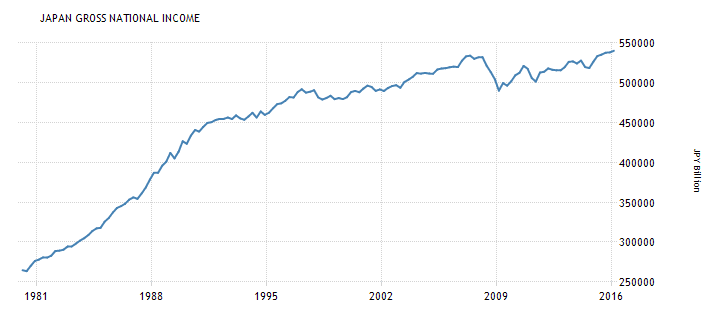GDI was around 270,000 in 1980

GDI was around 430,000 in 1989.

GDI was around 520,000 in 2007.

GDI is around 540,000 now.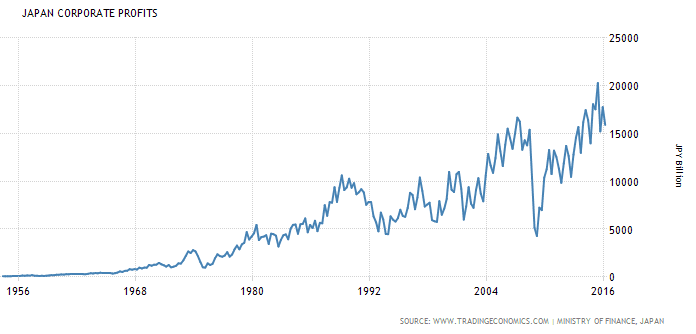Corporate profits peaked around 4,000 in 1980.

Corporate profits peaked around 10,000 in 1990.

Corporate profits peaked around 15,000 in 2007.

Corporate profits are around 17,000 now.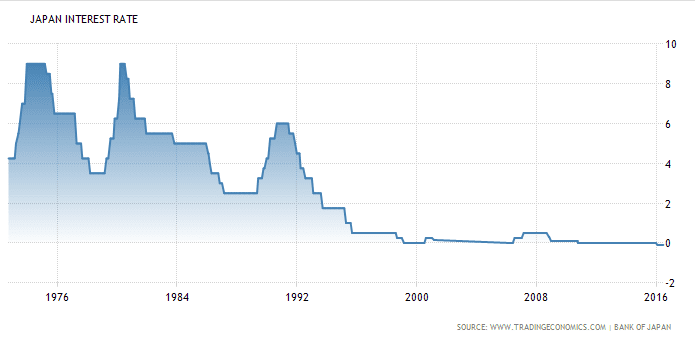Interest rate reported by Bank of Japan was around 5% in 1980.

Interest rate reported was around 4% in 1990.

Interest rate reported was around 0.25% in 2007.

Interest rate reported is around -0.1% now.

Let’s calculate some quick aggregate net corporate profit rates.

Net profit rate = Profits/GDI – interest rate

1980 = 4,000/270,000 – 5% = -3.5%%

1990 = 10,000/ 430,000 – 4% = -1.6%

2007 = 15,000/520,000 – 0.25% = 2.6%

Now = 17,000/540,000 – (- 0.1%) = 3.2%

The trend of increasing net profit rates is evident. Current rates are up 6% since 1980. That is similar to the United States.

It seems to me that Japan’s problem with low inflation stems from increasing net profit rates since the 1980’s… And taking interest rates into negative territory is not fixing that problem.

## Effect of High Net Profit Rate on Inflation & Interest Rates

I have been twittering a chart this morning which puts inflation alongside the net profit rate.

Net profit rate = Corporate profits/GDP – effective Fed rate

This measure is based on Keynes Marginal Efficiency of Capital. MEC.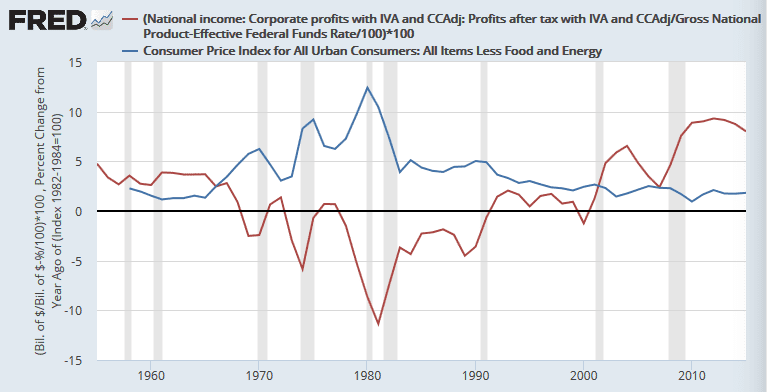As net profit rates went down in the 1970’s, firms felt pressure to raise prices to protect their profit rates. There was also increasing purchasing power of labor.

Then as net profit rates increased since the 1980’s, pressure to raise prices has decreased. Inflation has kept falling even to below Fed targets. Right now firms have negligible pressure to raise prices, especially since purchasing power has been eroded with low labor share.

Interest rates have gone low because inflation has gone low. So if we push the Fed rate into negative territory, we will try to increase net profit rates in order to increase investment. But the effect will only be to lessen the pressures to raise prices. Inflation will stay lower and lower.

So I do not buy the argument of secular stagnation. We have a situation where corporations have very high net profit rates and labor share is low.  The economic system is a supply-side, tricke-down, neo-feudalistic bad dream…

We really need to be raising the Fed rate to push net profit rates back down a bit… Make firms feel pressure to raise prices. Then labor will have to fight for more bargaining power… and things will get back to “normal”.

Comments Off on Effect of High Net Profit Rate on Inflation & Interest Rates | |

## Reflections on Anwar Shaikh: Equation for Corporate Profit Rate

A couple weeks ago, I found the work of Anwar Shaikh. Briefly, he has developed unique and better models to describe the economy, so that there is no need to use rational expectations, utility functions, monopoly power, and much more. He describes the economy as always developing around the central aspects of Real Competition and profitability.

He has numerous original equations and models.

He researches effective demand, as I do, so there is common ground. Our work shares similar factors used to determine effective demand. So I see areas where our work can blend toward better models.

This post is concerned with… Is there an equation to describe the profit maximization that takes place as the economy hits the effective demand limit?

In Shaikh’s book, Capitalism: Competiton, Conflict and Crisis,  he writes about profit rates, wage share, unemployment and capacity utilization.  These are factors that I use in my own work of effective demand. I was hoping to see an equation in his book to determine the aggregate profit rate of business as it is related to an effective demand limit, but he does not seem to have the effective demand limit nailed down yet. So he presents in a general way the idea that profit rates peak at an effective demand limit…

“So it becomes crucial to ascertain the conditions under which a rise in the real wage causes the normal rate of profit to fall.”

He is describing the rise in real wages,or labor share, to such a point as to make the aggregate profit rate fall. This would describe the effective demand limit.

So I set out to find an equation…

First, a brief explanation of effective demand as I see it.

Of aggregate national income, labor receives its labor share, or wage share as Anwar Shaikh calls it. Capitalists will then optimize the utilization of their capital to make profits off of the labor share. In the aggregate, capital does not want to make profits off of its own capital utilization. That would make profit rates decline. Labor share becomes the measure by which capital in the aggregate can determine its maximum profit rate.

Think of it this way… In general…

Labor share > utilization of capital … in order to insure increasing profit rates.

The portion of income from production that goes to labor must be greater than the portion of capital used for production. If utilization rates of capital in the aggregate supersede the effective labor share rate, then capital must consume its own income for production and capital profits will decline by eating themselves.

But not all of labor works… A certain % of labor is employed. So the true proportional share going to labor is found by…

Labor share/employment

Employment = (1 – unemployment)

Anwar Shaikh uses this measure of employment, (1 – unemployment), quite a bit in his work as I do.

From my work, the effective demand limit that labor share establishes upon utilization rates of capital is found in the central tendency of labor share within the cycles of capacity utilization. Anwar Shaikh is a proponent of central tendencies.

The equation from the central tendency to show the effective demand limit upon utilization rates of capital is…

Effective labor share = Labor share * 0.76

This is similar to Anwar Shaikh’s concept of a normal capacity utilization rate around which capacity utilization gravitates. I can offer Anwar Shaikh my equation using labor share to determine this normal rate.

So now the ideas come together in this equation.

Effective labor share/employment = Proportional effective labor share/100% employment

Let’s say for example that effective labor share is 75% and employment 95%. Then the left side of the equation says the 95% employed workers are receiving proportionally 79% labor share.  The labor share is given to only 95% of the labor force, not the whole labor force. If 100% of the labor force was employed, they would receive 79% of the income at the current production.

Proportional effective labor share = 79%

This 79% now becomes the limit threshold upon capital utilization. If capacity utilization were to rise above this limit, profit rates fpr capital income would start to fall. Here is a graph from my work to show that profit rates peak when capacity utilization is hitting the Proportional effective labor share limit. (1967 to 2015, years left out on x-axis.)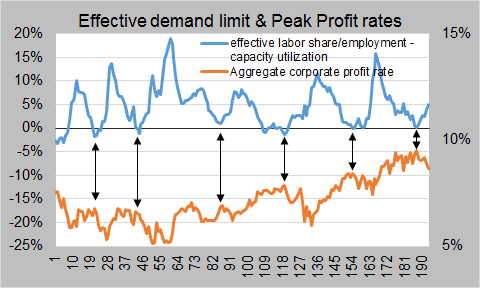Ok now… Can the same variables be used to estimate the profit rate itself? Yes…

Here is the equation which is built upon my cobra equation

Corporate profit rate = b * (2*(cap + emp + T2*els) – 3*(T2 + els))/2*els

cap = capacity utilization
emp = (1 – unemployment rate)
T = cap * emp
els = effective labor share
b = coefficient, 0.352 for United States

The equation uses just one simple variable coefficient (b). It is designed to give decreasing returns to utilization at the effective demand limit. Here is a graph of annual data since 1969… (data on profit rate)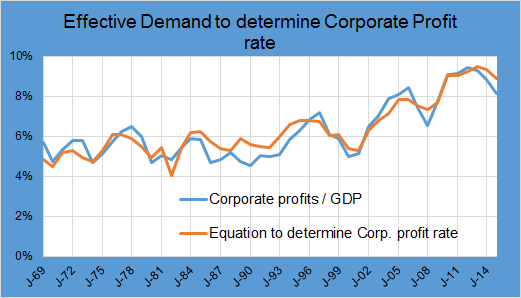The lines have a 93.6% correlation. They have the same average of 7.9%. The standard deviation of the difference between the two lines is just 0.5%. It is a good fit.

Here is what the equation looks like in 3-dimensional space with an effective labor share of 75%. It is like a cobra. (link to graph site)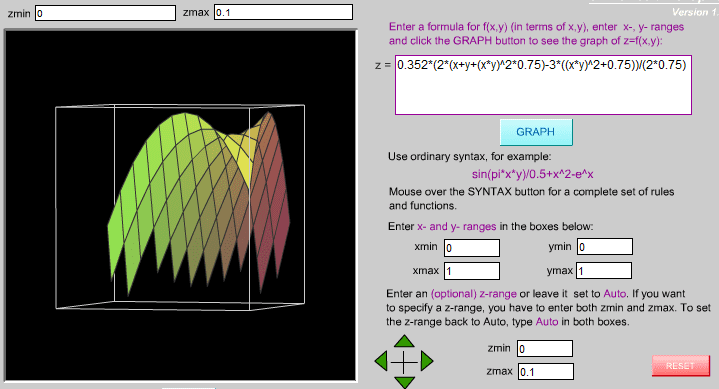The x and y axes are for capacity utilization and employment from 0% to 100%. The z-vertical has a range from 0% to 10% profit rate in the picture. Profit rates climb upwards on the back of the cobra increasing profits as they go.

So as Anwar Shaikh wrote…

“So it becomes crucial to ascertain the conditions under which a rise in the real wage causes the normal rate of profit to fall.”

The equation describes the conditions. It shows that as labor share rises, or as real wages rise faster than productivity, the aggregate profit rate falls. That is what we have seen since 2014. Labor share has risen 4% since profit rates peaked. It is a common dynamic at the end of a business cycle.

and Yes… we are heading toward an economic contraction… the dynamics of the equation above are pointing in that direction.

## Economic Puzzle for Discussion

A miner borrows \$100 for digging equipment. The bank loans him the money. He buys the equipment. He goes into the mountains, digs and brings back a chunk of gold worth \$10,000. He sells the chunk of gold to a jewelry maker.

How might the variables in this equation be changed?

M * V = P * Q

The current values are…

M, money supply = 500,000
V, velocity of money = 2
P, price level = 1
Q, gross domestic production = 1,000,000

## Instead of 4% inflation target, set “net profit rate” target at 4%

The debate over whether the Fisher Effect is real has languished over the past year. It is hard to prove, and even I lost hope in it. However, I may have found the mechanism to make it work.

First, look at this graph of the net profit rate plotted with core inflation since 1958… Chart updated to show “Sweet Spot of Fisher Effect”.A recently very high net profit rate of 9% led to a very low inflation rate according to the graph. Firms had little pressure to raise prices with such high net profit rates.

So the key would be to lower the net profit rate to a sweet spot of 3% to 6%. Then firms would have more reason in the aggregate to raise prices to net protect profit rates. Then the central tendency of core inflation would rise to 2% according to the graph.

How could we lower the net profit rate? Drum roll please… raise the Fed rate.

So raising the Fed rate while we are on the right side of the sweet spot would raise core inflation. If we were on the left side of the sweet spot, the economy would contract away to the left from the sweet spot as the net profit rate goes too low. So the Fisher Effect could only work properly (raising the Fed rate to raise inflation) if the net profit rate puts core inflation on the right side of the core inflation target.

The reason why the Fisher Effect has never been seen before is that we have been rarely, if at all, on the right side of the sweet spot since 1958. Net profit rate only topped 4% in 2003 and 2004 when it reached 6%. Since 2009, it has been over 8%!

So instead of a 4% inflation target, it could be better for the Fed to shoot for a 4% target in the net profit rate.

## Nice graph to show why inflation is low

Watching the videos of Anwar Shaikh, He presented an idea relating inflation to the net profit rate.

Net profit rate = Corporate profit rate – effective Fed rate

His view is that inflation will occur when the profit rate is squeezed by the base Fed rate. When net profit rate is low or even negative, inflation tends to occur because low net profit rates hinder investment and economic growth, and an attempt to stimulate the economy would show up as inflation of prices instead of an increase in GDP. He used this reasoning to explain the stagflation of the 70’s.

So I went to FRED and made a quick graph… (link to data) (Annual data from 1958 to 2015)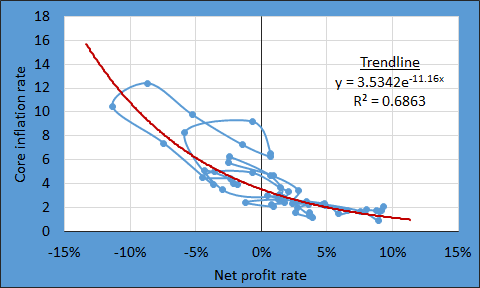The pattern is clear. When net profit rates are high, inflation must be low. In 2015, the annual data showed a high net profit rate of 8% with a low core inflation rate of 1.8%. When net profit rate reached -10% in the 1970s, inflation went high.

Let’s put the current core inflation rate into the trendline equation to estimate how the current net profit rate might be changing.

Core inflation = 2.2% = 3.534*e(-11.16*x)

Estimated net profit rate = x =   4.2%

So the net profit rate may have dropped from 8% to 5% in the last year. The rise in core inflation may be showing that net profit rates are falling. That would not be a good sign, because lower net profit rates slow down growth and investment which leads to an economic contraction.

## The Brilliance of Anwar Shaikh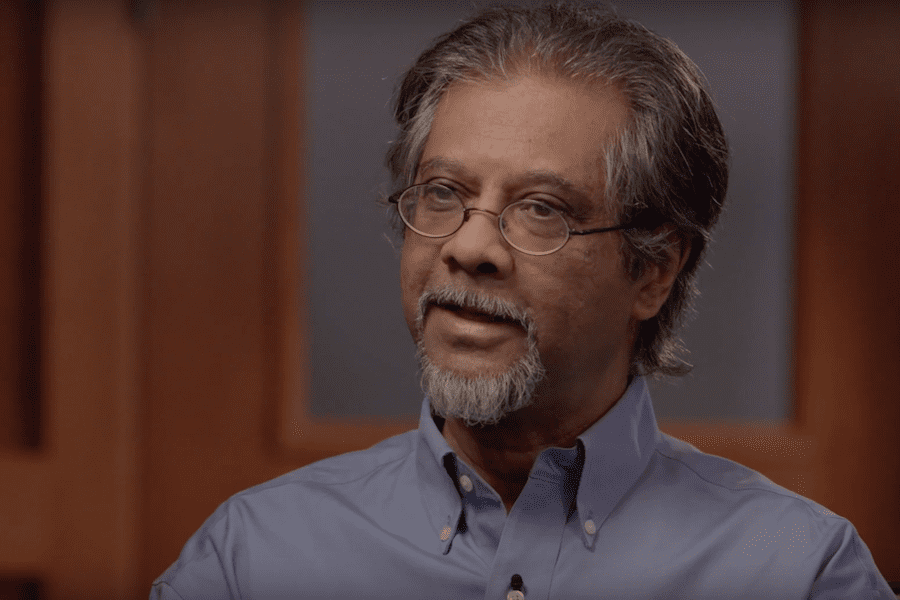Recently I found the work of Anwar Shaikh. His views on economics are the most insightful and thorough I have ever seen.  He is questioning and re-formulating economic theories through history. He is arriving at conclusions that are more realistic and integrative.

He should lead the way to how economics should be taught in the future. His free-thinking path is somewhat similar to that of Samuel Bowles and Joseph Stiglitz, but I think Shaikh goes beyond them to greater understanding of macroeconomics.

Here is a playlist from youtube for his 30-video lecture series. Each video is about 1.5 hours long. So there is a lot of brilliant material to go through.

link to playlist for Anwar Shaikh lectures

Here is an interview with INET that gives just small taste of his views. The video lectures present so much more.

Here is a link to get his 2016 book, Capitalism: Competition, Conflict & Crises

Why do I like his work?

• He says profit drives the business cycle.
• He says firms set prices.
• He does not believe in monopoly power, but rather the dynamics of capital intensity. Brilliant.
• He understands the labor struggle.
• He has great knowledge of economists through history and their work.
• He has seeks to explain Keynes’ Effective Demand with his theory of Real Competition and aggregate profitability. He is on the right path. Effective Demand is the area of my research. So I study his work to gain insights into my own work.
• He presents a view of firms trying to survive by either cutting prices or cutting capacity utilization depending on ease of entry/exit from an industry.
• And so much more…

I highly recommend the work Anwar Shaikh.

His lecture #8 might be a good place to start.

## Come on Banks, take notice of the AS-AD-ED Model

From feedback, I need to write a better post about the AS-ED model. Thanks for the feedback from Coberly, Arne and David at CEPR. (link to previous post)

This post presents what could be a huge breakthrough in understanding the business cycle.

Banks and central banks should take notice here.

### Model of Effective DemandFigure 1. This is a model for Aggregate supply, aggregate demand and effective demand.

People are familiar with aggregate supply and aggregate demand. In the AS-AD model, AS and AD always cross at the current real GDP and current core CPI, as shown at the red dot in the model. That red dot crossing point will move horizontally to the right as real GDP grows at a stable core inflation rate. Therefore, the AS curve in the model is horizontal to represent growing real GDP at a stable inflation.

### What is the Effective demand limit curve doing in the model?

Keynes described effective demand as the crossing point of aggregate demand and aggregate supply where aggregate profits are maximized. So as aggregate supply (real GDP) grows to the right, there comes a point where aggregate demand equals effective demand. In figure 1, that point is modeled around \$16,9 trillion. There profits would peak and the business cycle would begin a phase of deterioration into an economic contraction, unless other dynamics counteract the effects.

### What is the Basic Model for Effective Demand?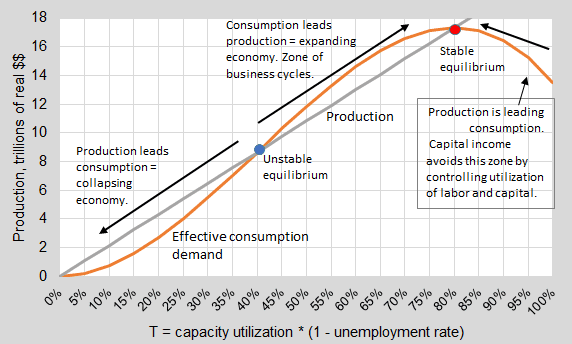Figure 2. Basic Model of Effective Demand upon Production.

In figure 2, the upsloping straight gray line is the AS curve from figure 1 related to utilization of labor and capital. As labor and capital get more utilized, real GDP production increases. In figure 3, actual data shows that real GDP does move along this straight line from the origin of x and y axes. (2ndQ 2010 to present, quarterly) This pattern is seen in other business cycles too.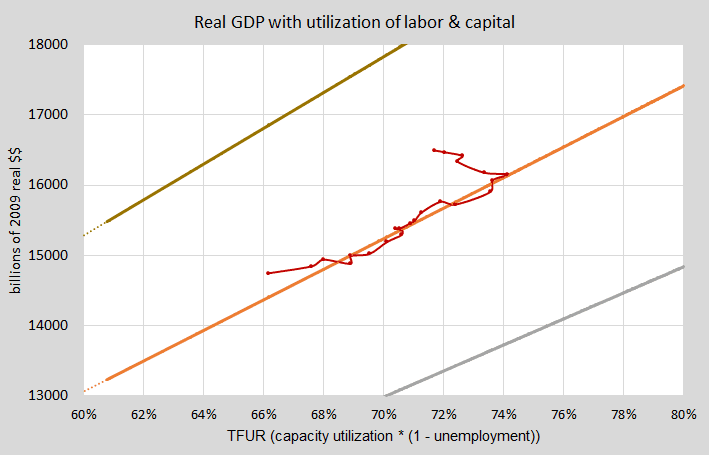Figure 3. Real GDP grows in line with (capacity utilization * (1 – unemployment rate))

In figure 2, the curving upsloping line is the effective demand limit curve. I formulated its equation from predator-prey dynamics in Population Ecology (link) and the work of Samuel Bowles on Lenders and Borrowers under Inequality. (Bowles, Samuel. The new economics of inequality and redistribution. Cambridge University Press, 2012. pp. 42-50)

In figure 2, where the effective demand curve crosses the production curve at the stable equilibrium is the effective demand limit.

The equation for the Effective Demand curve in figure 2 is…

Effective demand limit upon real GDP = rGDP*e*T/L* (1 – (1 – 1/e)*T/L)

rGDP = real GDP
T = capacity utilization * (1 – unemployment rate)
L = effective demand limit function (labor share index * 0.76)
e = 3

The peak of the profit cycle of production can be forecasted with this model since real GDP moves in a linear path and the effective demand limit is projected onto that path. Real GDP moves toward the projected effective demand limit.

### How did the model do during this business cycle?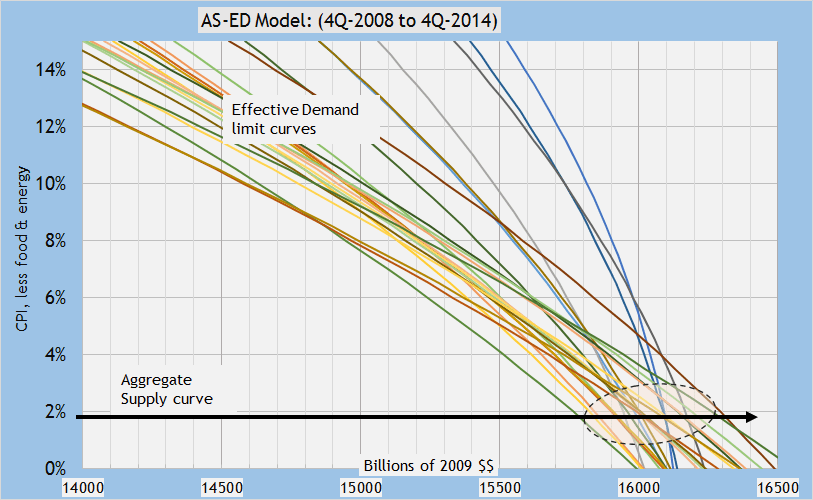Figure 4.  6 years of Effective Demand limit curves show great consistency. (25 quarters!) (Refer back to figure 1 for the ED curve in the AS-AD-ED model.

In figure 4, ALL, no cherry-picking, all the effective demand limit curves for 6 years crossed the AS curve in a tight zone. (see oval in figure 4) As real GDP started moving to the right on the AS curve from \$14.5 trillion at the end of 2008, the effective demand curves were waiting around \$16.0 trillion. As a confirmation, when real GDP hit about \$16.1 trillion, the effects of the effective demand limit appeared. The ED crossing points along the horizontal AS curve have a standard deviation of \$130 billion, Not too bad!

The model was a complete success in retrospect.

The equation for the Effective Demand limit curves in figure 4 is the same equation given for the ED curve in figure 2 but with L (effective demand limit function) replaced with (unit labor costs/(1+core CPI))… Now the equation can be plotted in AS-AD-ED space with core CPI on the y-axis.

• L = U/(1+C)
• U = L*((1+C)… This is how U is calculated for the equation below, since L and C are given in the model.

Effective demand limit upon real GDP = rGDP*e*T/(U/(1+C))* (1 – (1 – 1/e)*T/(U/(1+C)))

U = unit labor costs
C = core CPI %

### Conclusion

The tightness of the zone where the ED curves crosses the AS curve for 6 years is significant. The tightness reflects stability of the effective demand limit.

Profits peaked when real GDP hit the zone marked with an oval in 2014 just as Keynes would have forecasted with his explanation of effective demand.

The peak of the profit cycle, which drives the economy and business cycle, began to come into view 6 years in advance!

Really Folks, let that sink in…

That is a huge breakthrough!!!

• The Effective Demand model holds up very well in reality.
• The over-riding relationship between labor share and the utilization of labor and capital is governed by profits.
• The ability to forecast the effective demand limit upon profit cycles has huge value for banks and their investment cycles.